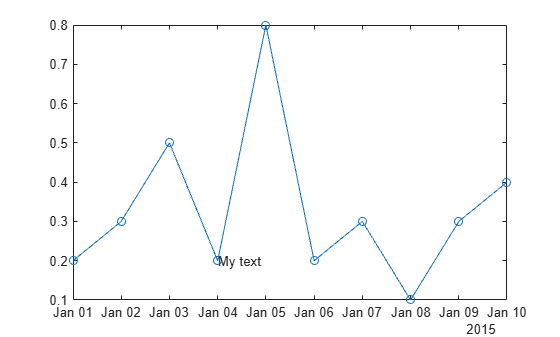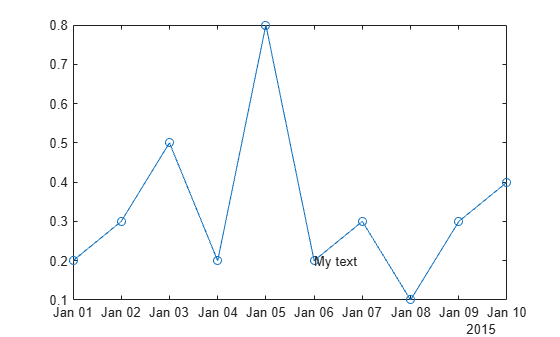# ruler2num

Convert data from specific ruler to numeric data

## Syntax

``num = ruler2num(data,ruler)``

## Description

example

````num = ruler2num(data,ruler)` converts the values in `data` to numeric values. The `ruler` input indicates the ruler associated with `data`, which determines how to map the values to the corresponding numeric values. The output `num` is the same size as the input `data`.```

## Examples

collapse all

Convert a date value to the equivalent numeric value in order to set the `Position` property of a text object.

Create a plot with dates along the x-axis. Add a text description to the fourth data point and return the text object.

```t = datetime(2015,1,1:10); y = [.2 .3 .5 .2 .8 .2 .3 .1 .3 .4]; plot(t,y,'-o') txt = text(t(4),y(4),'My text');```Change the position of the text to the sixth data point by setting the `Position` property of the text object. Since the `Position` property accepts only numeric values, convert the datetime value at the sixth data point to the equivalent numeric value.

```ax = gca; x6 = ruler2num(t(6),ax.XAxis); txt.Position = [x6 y(6)];```## Input Arguments

collapse all

Input array, specified as a scalar, vector, or matrix. If `data` is already numeric, then the output `num` is equal to the input `data`.

Ruler associated with the input data, specified as a `DatetimeRuler`, `DurationRuler`, `NumericRuler`, or `GeographicRuler` object. Specify the ruler object using the `XAxis`, `YAxis`, or `ZAxis` property of the `Axes` object. For example:

```ax = gca; num = ruler2num(data,ax.XAxis)```

If you specify a `NumericRuler` object and the input `data` is not numeric, then the output `num` is determined by:

`num = full(double(data))`

If you specify a `GeographicRuler` object, then the output `num` is equal to the input `data`.